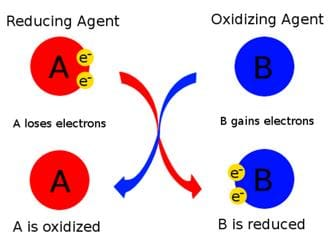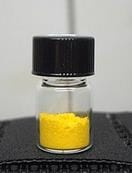Test: Chemical Reactions & Equations

# Test: Chemical Reactions & Equations

Test Description

## 25 Questions MCQ Test Science Class 10 | Test: Chemical Reactions & Equations

Test: Chemical Reactions & Equations for Class 10 2022 is part of Science Class 10 preparation. The Test: Chemical Reactions & Equations questions and answers have been prepared according to the Class 10 exam syllabus.The Test: Chemical Reactions & Equations MCQs are made for Class 10 2022 Exam. Find important definitions, questions, notes, meanings, examples, exercises, MCQs and online tests for Test: Chemical Reactions & Equations below.
Solutions of Test: Chemical Reactions & Equations questions in English are available as part of our Science Class 10 for Class 10 & Test: Chemical Reactions & Equations solutions in Hindi for Science Class 10 course. Download more important topics, notes, lectures and mock test series for Class 10 Exam by signing up for free. Attempt Test: Chemical Reactions & Equations | 25 questions in 25 minutes | Mock test for Class 10 preparation | Free important questions MCQ to study Science Class 10 for Class 10 Exam | Download free PDF with solutions
 1 Crore+ students have signed up on EduRev. Have you?
Test: Chemical Reactions & Equations - Question 1

### Which of the following is a combination reaction?

Detailed Solution for Test: Chemical Reactions & Equations - Question 1

Balanced Equation:
H2 + Cl2 → 2HCl2

The above reaction is an example of combination reaction because two different elements are combining to form a single compound.

Test: Chemical Reactions & Equations - Question 2

### Which of the following represent a double displacement reaction?

Detailed Solution for Test: Chemical Reactions & Equations - Question 2

It is a double displacement reaction. Na will displace Ag and combine with NO3 to form NaNO3. Ag will then combine with Cl and form AgCl. So the products of the reaction are AgCl and NaNO3.

Test: Chemical Reactions & Equations - Question 3

### Which of the following is a displacement reaction?

Detailed Solution for Test: Chemical Reactions & Equations - Question 3

Fe + CuSo4 → FeSO4 + Cu
is displacement reaction.
In these reaction iron (Fe) displace copper (Cu)
Hence, it is a displacement type of reaction.

(a) is a decomposition reaction.
(b) and (d) are neutralisation reactions.

Test: Chemical Reactions & Equations - Question 4

The reaction H2 + Cl2 → 2HCl is a :

Detailed Solution for Test: Chemical Reactions & Equations - Question 4

Balanced equation:
H2 + 2Cl2 → 2HCl2

The above reaction is an example of combination reaction.

Test: Chemical Reactions & Equations - Question 5

Which of the following is a decomposition reaction?

Detailed Solution for Test: Chemical Reactions & Equations - Question 5

A decomposition reaction occurs when one reactant breaks down into two or more products. Examples of decomposition reactions include the breakdown of hydrogen peroxide to water and oxygen, and the breakdown of water to hydrogen and oxygen.
2KClO3 → 2KCl + 3O2

Test: Chemical Reactions & Equations - Question 6

Which of the following statement is incorrect?

Detailed Solution for Test: Chemical Reactions & Equations - Question 6

An oxidizing agent oxidizes other substances and gains electrons; therefore, its oxidation state decreases. Hence, it gets reduced.Test: Chemical Reactions & Equations - Question 7

Which of the following is a combustion reaction?

Detailed Solution for Test: Chemical Reactions & Equations - Question 7

Oxidation reaction which produce heat and light is called combustion. Here, when we burn petrol in the presence of Oxygen, it produces heat and light which proves that it is a combination reaction.

So option C is the correct answer.

Test: Chemical Reactions & Equations - Question 8

A chemical equation is said to be balanced if number of

Detailed Solution for Test: Chemical Reactions & Equations - Question 8

Balanced chemical equation: A balanced equation is a chemical equation in which number of atoms of each element is equal on both sides of the equation i.e number of atoms of an element on reactant side = number of atoms of that element on the product side.

Test: Chemical Reactions & Equations - Question 9

Which statement is correct about the following reaction?
ZnO + CO → Zn + CO2

Detailed Solution for Test: Chemical Reactions & Equations - Question 9

ZnO(s) + CO(s) → Zn(s) + CO2(g)

• Since oxygen has combined with carbon, carbon is the one that is oxidized and ZnO is the oxidizing agent. Similarly carbon has taken the oxygen of ZnO, carbon is the reducing agent and ZnO is the one that has been reduced.
• Reducing agent-C
• Oxidizing agent-ZnO
• Oxidized-CO
• Reduced- ZnO
Test: Chemical Reactions & Equations - Question 10

The reaction C + O2  → CO2 + Heat is a:

Detailed Solution for Test: Chemical Reactions & Equations - Question 10

C + O2  → CO2 + Heat

• This is a Combination reaction because C and O2 are combining to produce one single compound CO2.
• This is also an Oxidation reaction because carbon is getting oxidized.
• This is also an exothermic reaction because in this reaction heat is getting released.
Test: Chemical Reactions & Equations - Question 11

Conversion of CaCO3 in to CaO as per following reaction is an example of
CaCO3 → CaO + CO2

Detailed Solution for Test: Chemical Reactions & Equations - Question 11
• In the given reaction, the compound breaks down into two simpler products.
• Calcium carbonate is decomposed to calcium oxide and carbon dioxide.
Test: Chemical Reactions & Equations - Question 12

Fe2O3 + 2Al → Al2O3 + 2Fe  This reaction is an example of

Detailed Solution for Test: Chemical Reactions & Equations - Question 12

Fe2O3 + 2Al → Al2O3 + 2Fe
This reaction is an example of a displacement reaction.
Here, Aluminum is more reactive metal than Fe. Therefore, Al will displace Fe from its oxide.

Test: Chemical Reactions & Equations - Question 13

In reaction SO2 + 2H2S → 2H2O + 3S, the reducing agent is

Detailed Solution for Test: Chemical Reactions & Equations - Question 13
• In the reaction SO2 is getting reduced to S as oxygen is being removed.
• Similarly, H2S is oxidised to S as hydrogen is removed.
• The species getting oxidised facilitates the reduction process and is the reducing agent.
• Hence, H2S is the reducing agent and Oxidising agent is SO2.
Test: Chemical Reactions & Equations - Question 14

An element X on exposure to moist air turns reddish-brown and a new compound Y is formed. The substance X and Y are

Detailed Solution for Test: Chemical Reactions & Equations - Question 14

An element X exposure to moist air, forms a reddish brown and a new compound Y is formed.
Fe + H2O +O2  →  Fe2 O3
This Fe2O3 is the reddish brown compound which is called rust.
So , X → Fe
Y →  Fe2O3

Test: Chemical Reactions & Equations - Question 15

What happens when dilute hydrochloric acid is added to iron fillings?

Detailed Solution for Test: Chemical Reactions & Equations - Question 15
• Hydrogen gas and iron chloride are produced.

Fe + HCl →  FeCl + H2

• The iron displaces hydrogen from hydrochloric acid to form iron (II) chloride & hydrogen gas. This is a single displacement reaction.
• Thus the answer is option (A) Hydrogen gas and iron chloride are produced.
Test: Chemical Reactions & Equations - Question 16

When Iron nails are added to an aquous solution of copper sulphate, a chemical change occurs, which of the following is NOT true about this reaction?

Detailed Solution for Test: Chemical Reactions & Equations - Question 16

Fe(s) + CuSO4​ ⟶ Cu(s) + FeSO4​

When an iron nail is dipped in copper sulphate solution, a brown coating of copper is formed on the surface of iron and the colour of copper sulphate solution changes from blue to light green. This reaction shows that iron is more reactive than copper as it displaces copper from its solution and iron passes into solution as Fe2+ ions and ferrous sulphate solution is formed.

Test: Chemical Reactions & Equations - Question 17

Some crystals of copper sulphate were dissolved in water. The colour of the solution obtained would be

Detailed Solution for Test: Chemical Reactions & Equations - Question 17

Copper sulphate aqueous solution is blue in colour .

Test: Chemical Reactions & Equations - Question 18

The colour of zinc metal is

Detailed Solution for Test: Chemical Reactions & Equations - Question 18

Zinc is a bluish-silver, lustrous metal that tarnishes in moist air, producing a layer of carbonate. Zinc reacts with both acids and alkalis. Zinc is an essential trace element for animals and plants.

Test: Chemical Reactions & Equations - Question 19

Which one of the following will be required to identify the gas evolved when dilute HCl reacts with zinc metal?

Detailed Solution for Test: Chemical Reactions & Equations - Question 19

A burning splinter since hydrogen gas burns with a pop sound.

Zinc being an active metal readily reacts with hydrochloric acid at room temperature to form soluble zinc chloride and hydrogen.

Zn + 2HCl → ZnCl2 + H2.

Test: Chemical Reactions & Equations - Question 20

Can we store CuSO4 in iron container?

Detailed Solution for Test: Chemical Reactions & Equations - Question 20
• No we can't store copper sulphate in an iron vessel.
• Iron is more reactive than copper and it will displace copper from its sulphate solution.
• If we store it, the iron pot will get destroyed.
Test: Chemical Reactions & Equations - Question 21

Which of the following is the INCORRECT method of preventing rusting?

Detailed Solution for Test: Chemical Reactions & Equations - Question 21

To prevent rusting:

• The iron articles should be painted, the machine parts should be oiled or greased, galvanized iron pipes are used for water supply.
• But ( iron articles should be covered with plastic) is the incorrect method of preventing rusting.
Test: Chemical Reactions & Equations - Question 22

PbI2 is

Detailed Solution for Test: Chemical Reactions & Equations - Question 22

It is a bright yellow odourless crystalline solid, that becomes orange and red when heated. It was formerly called plumbous iodide.Test: Chemical Reactions & Equations - Question 23

The element or compound which undergoes reduction is

Detailed Solution for Test: Chemical Reactions & Equations - Question 23

An oxidizing agent, or oxidant, gains electrons and is reduced in a chemical reaction. Also known as the electron acceptor.

Test: Chemical Reactions & Equations - Question 24

Which of the following is not an example of chemical change?

Detailed Solution for Test: Chemical Reactions & Equations - Question 24

The process of changing water vapour into water is called condensation. It is not a chemical reaction.

When milk converts in curd the process is irreversible and a new substance with different properties is formed hence it is an example of a chemical change.

Rusting of iron is also a chemical change.

Test: Chemical Reactions & Equations - Question 25

ANa + BH2O → CNaOH + DH2. What would come in place of A , B, C and D to balance the equation?

Detailed Solution for Test: Chemical Reactions & Equations - Question 25

2Na + 2H2O → 2NaOH + H2

A balanced equation is an equation for a chemical reaction in which the number of atoms for each element in the reaction and the total charge are the same for both the reactants and the products.

## Science Class 10

83 videos|341 docs|162 tests
 Use Code STAYHOME200 and get INR 200 additional OFF Use Coupon Code
Information about Test: Chemical Reactions & Equations Page
In this test you can find the Exam questions for Test: Chemical Reactions & Equations solved & explained in the simplest way possible. Besides giving Questions and answers for Test: Chemical Reactions & Equations, EduRev gives you an ample number of Online tests for practice

## Science Class 10

83 videos|341 docs|162 tests